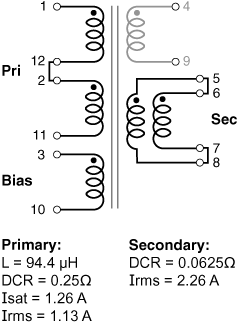## Website-Einstellungen

##### Währungseinstellungen
Produkte
HF
EMI
Informationsmaterial
Produktdokumentation
Handhabung und Verarbeitung
Modelle und Layout-Tools
Kurse/Schulung
Allgemein
Video-Bibliothek
Cx Family Common Mode Chokes
0402CT Low Profile Chip Inductors
XAL7050 High-inductance Shielded Power Inductors
XGL4020 Ultra-low DCR Power Inductors
Quality
Qualitätszertifizierung
Materialzertifizierung
Sicherheitszertifizierung
Zuverlässigkeit
Handhabung / Verarbeitung
Über
Über
Jobs
Standorte
Informationsmaterial

# Application example

for Hexa-Path – Flyback

<- Back

## Formulas used to calculate electrical characteristics

Connecting windings in series:

• Inductance = Inductance(table) × (number of windings)2
• DCR = DCR(table) × number of windings
• Isat = (Isat(table) × 6) ÷ number of windings connected in series
• Irms = Irms(table)

Connecting windings in parallel:

• Inductance = Inductance(table)
• DCR = 1 ÷ [number of windings × (1 ÷ DCR(table))]
• Isat = (Isat(table) × 6) ÷ number of windings connected in series
• Irms = Irms(table) × number of windings

### Create a 13 Watt 2:1:1 flyback transformer with a bias winding

Choose HPH3-0138L
Vin = 36 – 57 Vdc; Vout = 12 V, 1.1 A

Part number Inductance (µH) Percent Tolerance DCR max (Ω) Volt-time product (V-µs) Peak energy storage (µJ) Isat (A) Irms (A)
HPH3-0138L 23.6 20 0.125 43.9 1.457 0.42 1.13

Connecting primary windings in series:
When primary windings (Wpri) are connected in series, inductance increases, energy storage and Irms remain the same, but DCR increases and Isat decreases.

Example: For HPH3-0138L, connect two primary windings in series:
• Inductance = Inductance(table) × Wpri2 = 23.6 × 22 = 94.4 μH
• DCR = DCR(table) × Wpri = 0.125 × 2 = 0.25 Ohms
• Isat = (Isat(table) × 6) ÷ Wpri = (0.42 × 6) ÷ 2 = 1.26 A
• Irms = Irms(table) = 1.13Connecting secondary windings in parallel:
When secondary windings (Wsec) are connected in parallel, DCR decreases and Irms increases.

Example: For HPH3-0083L, connect two secondary windings in parallel:
• DCR = 1 ÷ [Wsec × (1 ÷ DCR(table))] = 1 ÷ [(2 × (1 ÷ 0.125)] = 0.0625 Ohms
• Irms = Irms(table) × Wsec = 1.13 × 2 = 2.26 A